# Factors

In this section, you will learn about factors in multiplication, algebra factors, as well as the factor definition to strengthen your concepts in factors.

Check-out the interactive simulations to know more about the lesson and try your hand at solving a few interesting practice questions at the end of the page.

But before we get into the details, let us understand the factor definition. A factor is a Latin word, and it means "a doer" or "a maker" or "a performer."

A factor of a number in math is a number that divides the given number.

Hence, a factor is nothing but a divisor of the given number.

## Lesson Plan

 1 What Are Factors? 2 Tips and Tricks 3 Solved Examples on Factors 4 Challenging Questions on Factors 5 Interactive Questions on Factors

## What Are Factors?

### Factor Definition

A factor is a number that divides the given number without any remainder.

### Examples of Finding Factors of a Number

Let us see how to find the factors of 12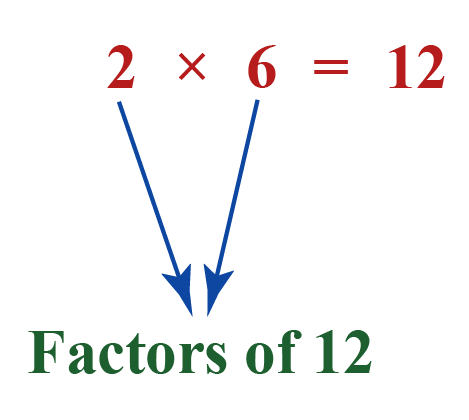2 and 6 are some of the factors of 12 as each of them divides 12 without any remainder.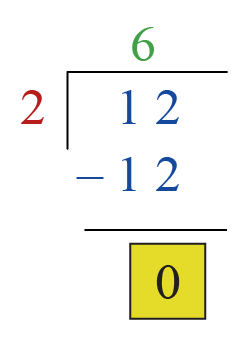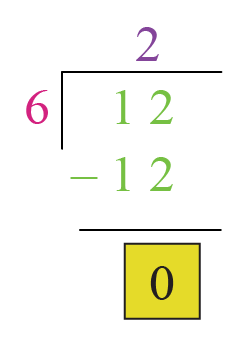Are there any factors of 12 apart from 2 and 6?

Yes, 1, 3, 4, and 12 also divide 12 along with 2 and 6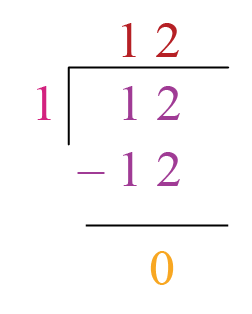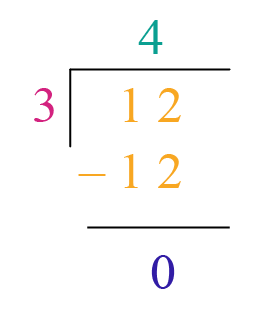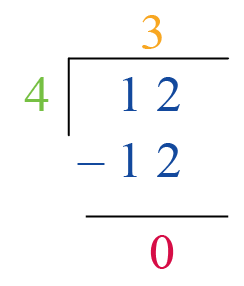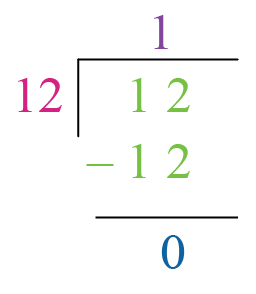Thus, the factors of 12 are 1, 2, 3, 4, 6, and 12

Apart from these, there can be negative factors of 12 as well such as -1, -2, -3, -4, -6, and -12

## Properties of Factors

The properties of factors are:

• The number of factors of a number is finite.
• A factor of a number is always less than or equal to the given number.
• Every number except 0 and 1 has at least two factors, 1 and itself.
• Division and multiplication are the operations that are used in finding the factors.

## How to Find Factors?

We can use both "Division" and "Multiplication" to find the factors.

### Factors in Division

To find the factors of a number using division:

• Find all the numbers less than or equal to the given number.
• Divide the given number by each of the numbers.
• The divisors that give the remainder to be 0 are the factors of the number
(by the definition of a factor of a number)

Example:

Find the positive factors of 6 using division.

Solution:

The positive numbers that are less than or equal to 6 are 1, 2, 3, 4, 5, and 6

Let us divide 6 by each of these numbers.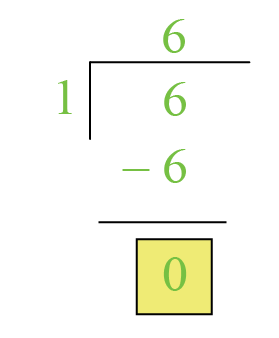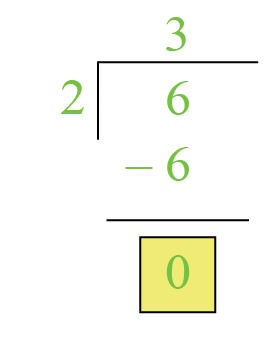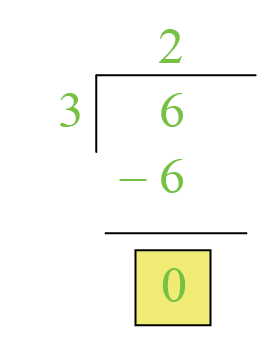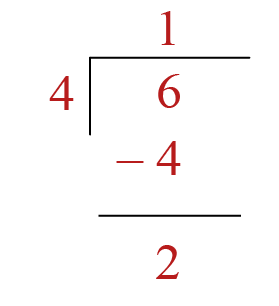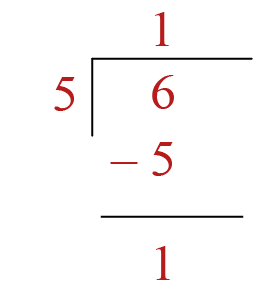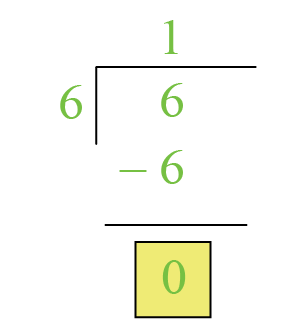We can observe that the divisors 1, 2, 3, and, 6 give zero as the remainder.

 Thus, factors of 6 are: 1, 2, 3, and 6

### Factors in Multiplication

To find the factors using the multiplication:

• Write the given number as the product of two numbers in different possible ways.

• All the numbers that are involved in all these products are the factors of the given number.

Example:

Find the positive factors of 24 using multiplication.

Solution:

We will write 24 as the product of two numbers in multiple ways.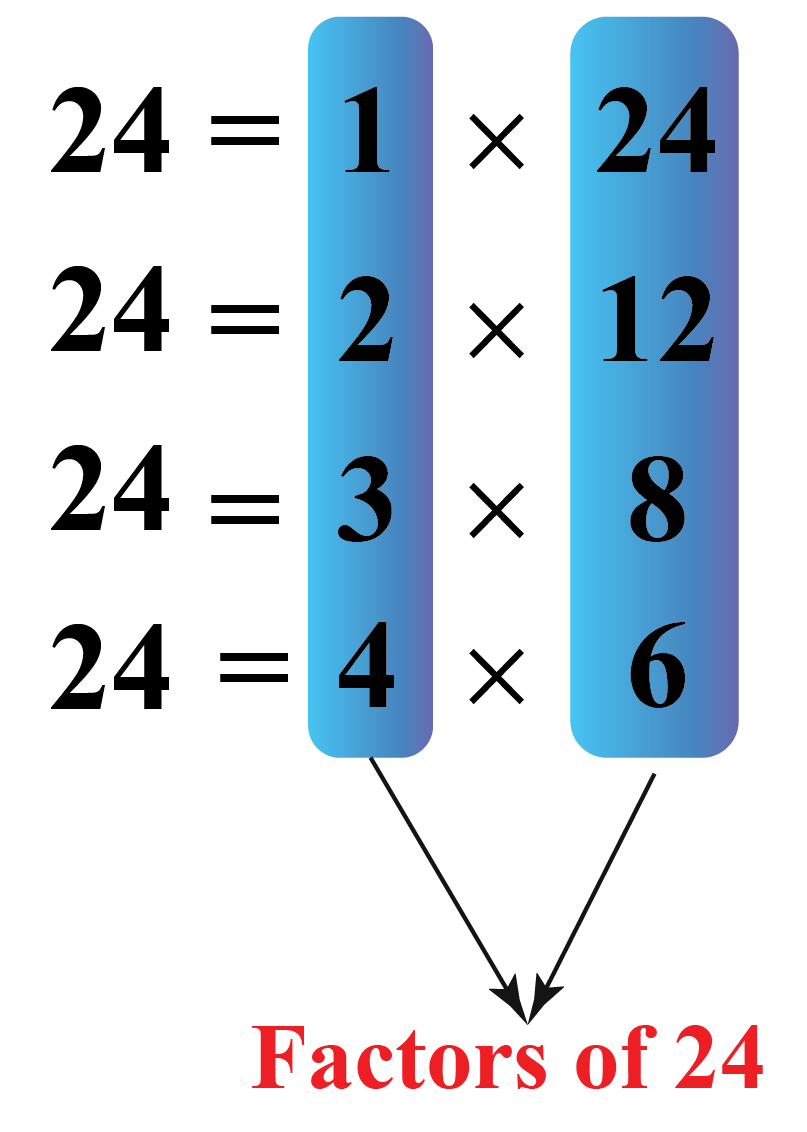All the numbers that are involved in these products are the factors of the given number (by the definition of a factor of a number)

 Thus, the factors of 24 are: 1, 2, 3, 4, 6, 8, 12, and 24Tips and Tricks
1. To find the factors, we can apply the divisibility rules.
2. The numbers greater than half of a given number (except the number itself) CANNOT be the factors of the given number.
For example, while finding the factors of $$50$$, it is sufficient to check for the numbers from $$1$$ to $$25$$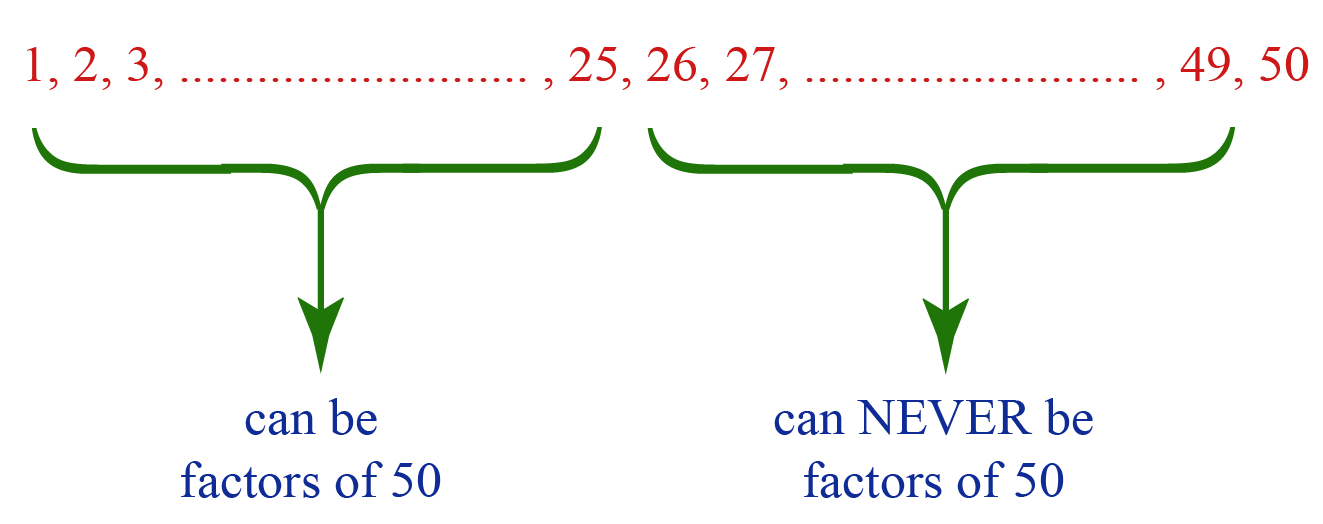## Factors Calculator

Here is the "Factors Calculator."

We can enter any number here.

Then the calculator will show its factors using multiplication with a step-by-step explanation.

## Finding Number of Factors

We can find the number of factors of a given number using the following steps.

• Find its prime factorization, i.e. express it as the product of primes.
• Write the prime factorization in the exponent form.
• Add $$1$$ to each of the exponents.
• Multiply all the resultant numbers.
• This product would give the number of factors of the given number.

Example:

Find the number of factors of the number 108

Solution:

Perform prime factorization of the number 108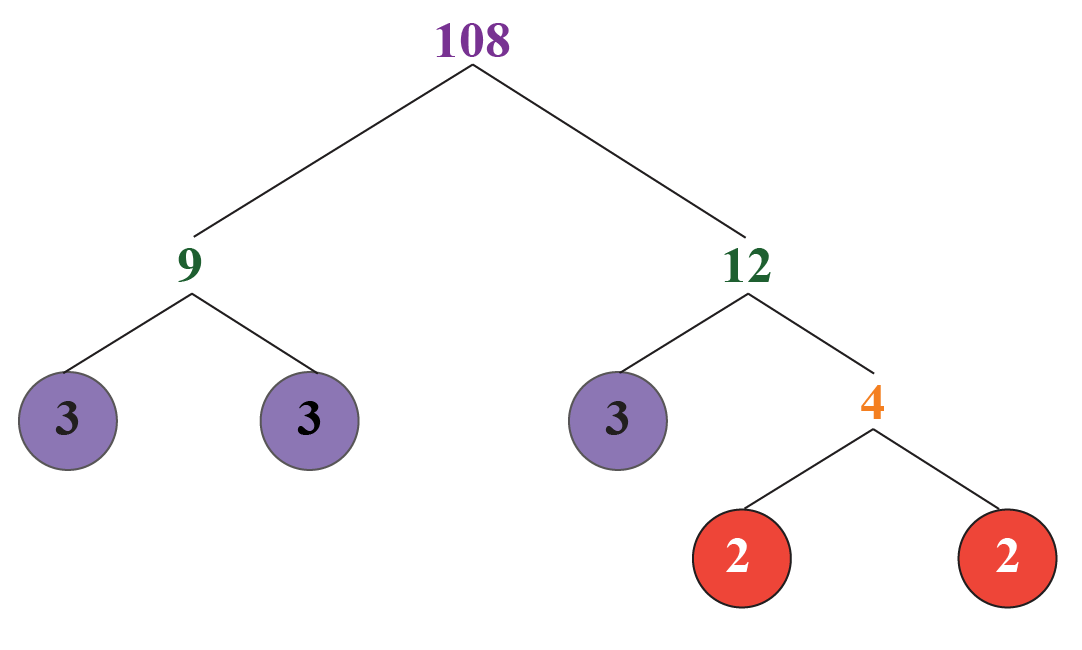Thus,

$108 = 2 \times 2 \times 3 \times 3 \times 3$

Write this prime factorization in the exponent form.

$108 = 2^2 \times 3^3$

Add $$1$$ to each of the exponents, $$2 \text{ and } 3$$, here.

Then:

$2+1=3\\3+1=4$

Multiply these numbers:

$3 \times 4 = 12$

 Thus, Number of factors of 108 is 12

The actual factors of 108 are 1, 2, 3, 4, 6, 9, 12, 18, 27, 36, 54, and 108

Here, 108 has 12 factors and hence our above answer is correct.

## Algebra-Factors

We saw what are the factors of a number.

Factors do exist for an algebraic expression as well.

For example, the factors of $$6x$$ are $$1,2,3,6, x, 2x,3x,$$ and $$6x$$.

There are different types of procedures to find factors in Algebra.

Some of them are as follows:

Click on the above links to learn each of them in detail.

## Solved Examples

 Example 1

Find the positive factors of 64

Solution

Half of the given number is 32

To find the factors of 64, it is sufficient to check the numbers from 1 to 32 whether they give the remainder zero when we divide 64 by them.

We can observe that the numbers 1, 2, 4, 8, 16, and 32 give the remainder 0 when we divide 64 by each of them.

Thus, the factors of 64 are:

 1, 2, 4, 8, 16, and 32
 Example 2

Which of the following statement(s) is/are true?

1. The factor of a number can be greater than the number.

2.  Some numbers can have infinite number of factors.

Solution

1. The first statement, "The factor of a number can be greater than the number," is FALSE.

We know that factors are the divisors of the number that leave $$0$$ as the remainder.

Hence, they are always less than the number.

1. The second statement, "Some numbers can have infinite number of factors," is FALSE.

The number of factors of a number is finite.

 Example 3

Find the number of factors of 1620

Solution

Find the prime factorization of 1620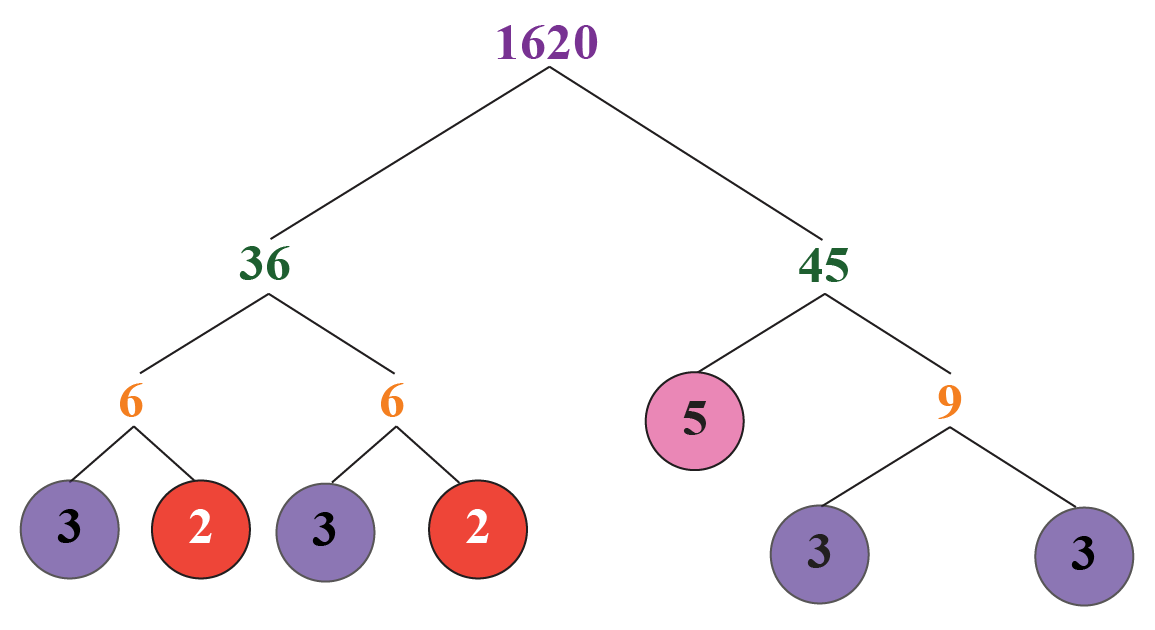Thus,

$1620 = 2^{2} \times 3^{4} \times 5^{1}$

Add 1 to each of the exponents.

We get:

$2+1=3\\4+1=5\\1+1=2$

Find the product of all these numbers:

$3 \times 5 \times 2 = 30$

 Thus, Number of factors of 1620 is 30Challenging Questions
1. Brandon has to split 36 students in his class into different groups with an equal number of students in each. All students cannot be in one group and each group should have more than one student. In how many ways can he form these groups

## Interactive Questions

Here are few activities for you to practice. Select/Type your answer and click the "Check Answer" button to see the result.

## Important Topics

Given below are the list of topics that are closely connected to Factors. These topics will also give you a glimpse of how such concepts are covered in Cuemath.

•

## Let's Summarize

We hope you enjoyed learning about Factors with the simulations and practice questions. Now you will be able to easily solve problems on factor definition, factors in multiplication and algebra factors.

At Cuemath, our team of math experts is dedicated to making learning fun for our favorite readers, the students!

Through an interactive and engaging learning-teaching-learning approach, the teachers explore all angles of a topic.

Be it worksheets, online classes, doubt sessions, or any other form of relation, it’s the logical thinking and smart learning approach that we, at Cuemath, believe in.

## 1. How do you factor equations?

We cannot actually factor equations, but we can factor expressions.

Factoring an expression is writing it as the product of two or more expressions.

For example:

$3x^2+6x = 3x(x+2)$

## 2. What is prime factorization?

The prime factorization of a number is writing it as the product of two or more prime numbers.

For example: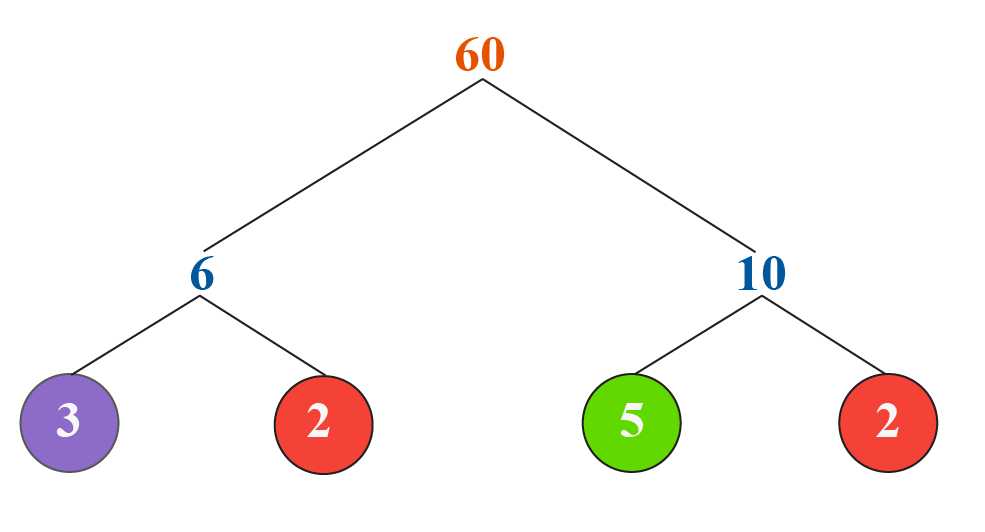The prime factorization of 60 = $$2^2 \times 3 \times 5$$.

## 3.  How to find number of factors?

We can find the number of factors of a given number using the following steps.

• Find its prime factorization, i.e. express it as the product of primes.
• Write the prime factorization in the exponent form.
• Add $$1$$ to each of the exponents.
• Multiply all the resultant numbers.
• This product would give the number of factors of the given number.

Divisibility
Divisibility
Factors and Multiples
Factors and Multiples
Factors, Multiples, and Primes
Factors, Multiples, and Primes
Divisibility